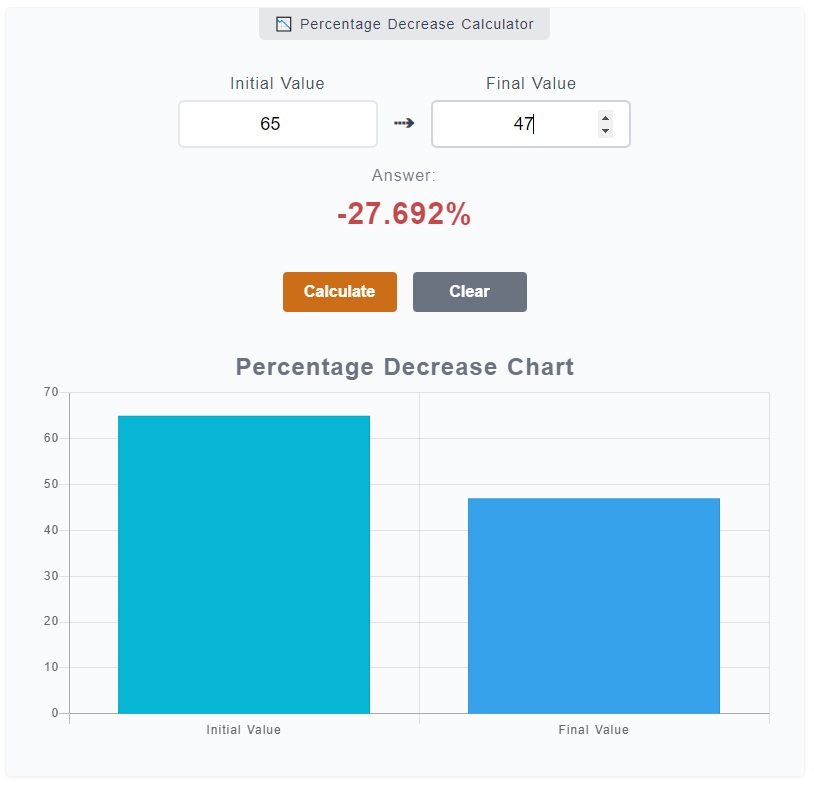# Percentage Decrease Calculator

The Percentage Decrease Calculator is used to measure the decrease of value from an initial to its final value in terms of percentage. On other words, you can use this calculator to measure the decline of value from \$50 which now becomes \$15. You just need to input the values on the corresponding fields and press `Enter`. The result shall be displayed right away.

📉 Percentage Decrease Calculator

0%

## How to Use the Percentage Decrease Calculator

Regardless of you are computing for a decrease of stock value, you have traded down an item or you are comparing prices, this calculator simply gives you the relative percentage decrease.

The `Percentage Increase Calculator` is quite a simple tool that you can see right away the input fields with corresponding labels. You can just enter numbers in them and click the `Calculate` button to get your answer. However, here's a step by step guide to its usage.1. Step 1

On the calculator, there are two (2) input fields - initial value and final value. On the initial value, enter the number from its starting value. Enter the final value on the corresponding field as well. For instance, you want to know the percentage decrease from 65 to 47. Enter `65` on `Initial Value` input field and `47` to the `Final Value` input field.

2. Step 2

To get your results, you have two (2) options. You can press the `Enter` key on your keyboard or you can click on the `Calculate` button. To clear the input fields, result and percentage decrease chart, you just need to click the `Clear` button.